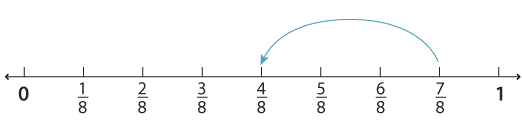### Subtraction of fractions with the same denominator

The subtraction of two fractions with the same denominators can be thought of as steps on the number line.

To subtract $$\dfrac{3}{8} \text{from} \dfrac{7}{8}$$ we start at $$\dfrac{7}{8}$$ and take a step of $$\dfrac{3}{8} \text{to get} \dfrac{4}{8}$$.Detailed description

We write:

\begin{align}\dfrac{7}{8} − \dfrac{3}{8} &= \dfrac{4}{8} \\&= \dfrac{1}{2} \ \text{(simplify)}.\end{align}